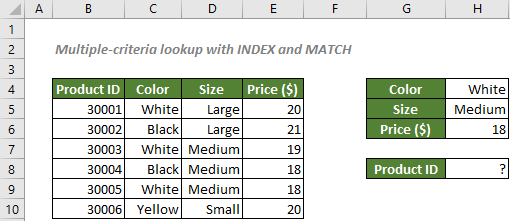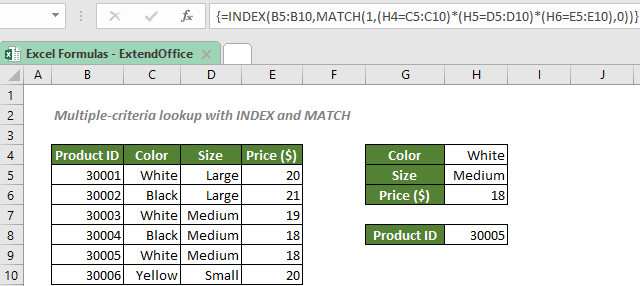Note: The other languages of the website are Google-translated. Back to English

## Multiple-criteria lookup with INDEX and MATCH

When dealing with a big database in an Excel spreadsheet with several columns and row captions, it’s always tricky to find something that meets multiple criteria. In this case, you can use an array formula with the INDEX and MATCH functions.#### How to perform a lookup with multiple criteria?

To find out the product that is white and medium-sized with a price of \$18 as shown in the above picture, you can take the advantage of the boolean logic to generate an array of 1s and 0s to show the rows that meet the criteria. The MATCH function will then find the position of the first row that meet all the criteria. After that, INDEX will find the corresponding product ID on the same row.

#### Generic syntax

=INDEX(return_range,MATCH(1,(criteria_value1=criteria_range1*criteria_value2=criteria_range2*(…),0))

√ Note: This is an array formula that requires you to enter with Ctrl + Shift + Enter.

• return_range: The range where you want the combination formula to return the product ID from. Here refers to the product ID range.
• criteria_value: The criteria used to locate the position of the product ID. Here refers to the values in the cells H4, H5 and H6.
• criteria_range: The corresponding ranges where the criteria_values are listed. Here refers to the color, size and price ranges.
• match_type 0: Forces MATCH to find the first value that exactly equals to the lookup_value.

To find the product that is white and medium-sized with a price of \$18, please copy or enter the formula below in the cell H8, and press Ctrl + Shift + Enter to get the result:

=INDEX(B5:B10,MATCH(1,("White"=C5:C10)*("Medium"=D5:D10)*(18=E5:E10),0))

Or, use a cell reference to make the formula dynamic:

=INDEX(B5:B10,MATCH(1,(H4=C5:C10)*(H5=D5:D10)*(H6=E5:E10),0))#### Explanation of the formula

=INDEX(B5:B10,MATCH(1,(H4=C5:C10)*(H5=D5:D10)*(H6=E5:E10),0))

• (H4=C5:C10)*(H5=D5:D10)*(H6=E5:E10): The formula compares the color in the cell H4 against all the colors in the range C5:C10; compares the size in H5 against all sizes in D5:D10; compares the price in H6 against all prices in E5:E10. The initial result is like this:
{TRUE;FALSE;TRUE;FALSE;TRUE;FALSE}*{FALSE;FALSE;TRUE;TRUE;TRUE;FALSE}*{FALSE;FALSE;FALSE;TRUE;TRUE;FALSE}.
The multiplication will transform the TRUEs and FALSEs to 1s and 0s:
{1;0;1;0;1;0}*{0;0;1;1;1;0}*{0;0;0;1;1;0}.
After multiplication, we will have a single array like this:
{0;0;0;0;1;0}.
• MATCH(1,(H4=C5:C10)*(H5=D5:D10)*(H6=E5:E10),0) = MATCH(1,{0;0;0;0;1;0},0): The match_type 0 asks the MATCH function to find the exact match. The function will then return the position of 1 in the array {0;0;0;0;1;0}, which is 5.
• INDEX(B5:B10,MATCH(1,(H4=C5:C10)*(H5=D5:D10)*(H6=E5:E10),0)) = INDEX(B5:B10,5): The INDEX function returns the 5th value in the product ID range B5:B10, which is 30005.

#### Related functions

Excel INDEX function

The Excel INDEX function returns the displayed value based on a given position from a range or an array.

Excel MATCH function

The Excel MATCH function searches for a specific value in a range of cells, and returns the relative position of the value.

#### Related Formulas

Lookup closest match value with multiple criteria

In some cases, you may need to lookup the closest or approximate match value based on more than one criteria. With the combination of INDEX, MATCH and IF functions, you can quickly get it done in Excel.

Approximate match with INDEX and MATCH

There are times when we need to find approximate matches in Excel to evaluate employees' performance, grade students’ scores, calculate postage based on weight, etc. In this tutorial, we will talk about how to use the INDEX and MATCH functions to retrieve the results we need.

Lookup values from another worksheet or workbook

If you know how to use VLOOKUP function to search for values in a worksheet, vlookup values from another worksheet or workbook won’t be a problem for you. The tutorial will show you how to vlookup values from another worksheet in Excel.

### The Best Office Productivity Tools

#### Kutools for Excel - Helps You To Stand Out From Crowd

Would you like to complete your daily work quickly and perfectly? Kutools for Excel brings 300 powerful advanced features (Combine workbooks, sum by color, split cell contents, convert date, and so on...) and save 80% time for you.

• Designed for 1500 work scenarios, helps you solve 80% Excel problems.
• Reduce thousands of keyboard and mouse clicks every day, relieve your tired eyes and hands.
• Become an Excel expert in 3 minutes. No longer need to remember any painful formulas and VBA codes.
• 30-day unlimited free trial. 60-day money back guarantee. Free upgrade and support for 2 years.#### Office Tab - Enable Tabbed Reading and Editing in Microsoft Office (include Excel)

• One second to switch between dozens of open documents!
• Reduce hundreds of mouse clicks for you every day, say goodbye to mouse hand.
• Increases your productivity by 50% when viewing and editing multiple documents.
• Brings Efficient Tabs to Office (include Excel), Just Like Chrome, Firefox, And New Internet Explorer.